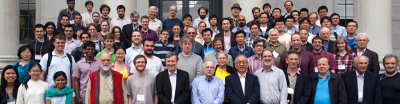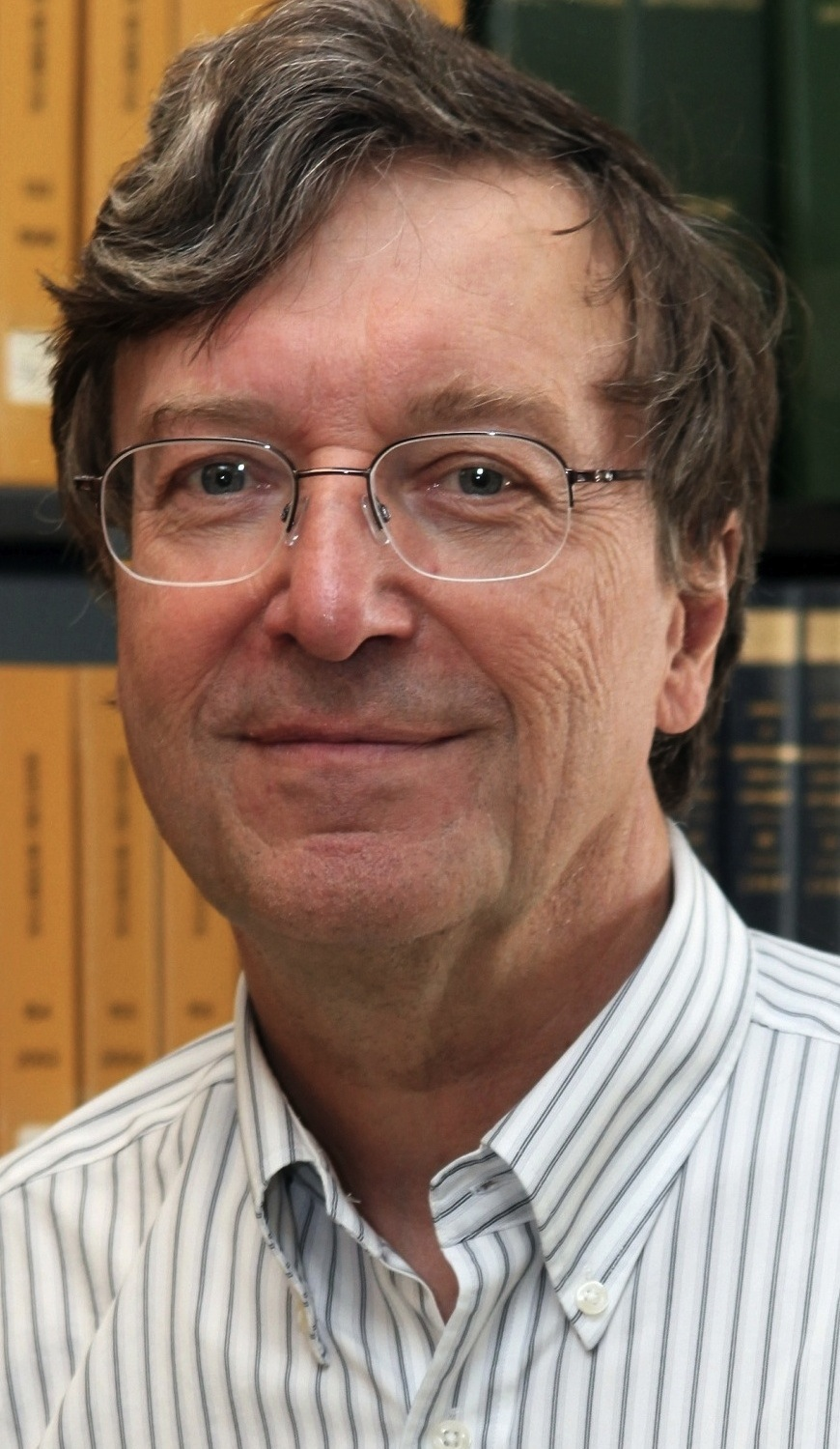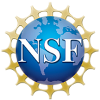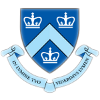Poster [PDF]
Photos I
Photos II
Open problems

Representation Theory, Automorphic Forms, and Complex GeometryA conference in honor of the 70th birthday of Wilfried Schmid

Harvard University, Science Center Hall C, May 20-23, 2013

Speakers include:

James Arthur (Toronto), Dan Barbasch (Cornell), Dennis Gaitsgory (Harvard), Benedict Gross (Harvard), Joe Harris (Harvard), David Kazhdan (Hebrew University), Xiaoqing Li (Buffalo), Alina Marian (Northeastern), Andrei Reznikov (Bar-Ilan), Peter Sarnak (Princeton), Diana Shelstad (Rutgers), Carlos Simpson (CNRS), Birgit Speh (Cornell), Kari Vilonen (Northwestern), David Vogan (MIT), Nolan Wallach (UCSD).

Organizing Committee:

Bill Casselman (British Columbia), Dorian Goldfeld (Columbia), Benedict Gross (Harvard), Mark Kisin (Harvard), Dragan Milicic (Utah), Stephen D. Miller (Rutgers), Peter E. Trapa (Utah), Shing-Tung Yau (Harvard).

Program

 Monday: 09:45 Opening remarks 10:00-11:00 Benedict Gross Why are p-adic groups more difficult than real groups? 11:30-12:30 David Kazhdan The existence of opers for classical groups. 14:30-15:30 Diana Shelstad Spectral questions in endoscopic transfer for real groups Slides [PDF] 16:00-17:00 Dennis Gaitsgory Verdier duality and geometric Langlands correspondence 18:00-19:30 Problem session led by Barry Mazur Tuesday: 09:30-10:30 Jim Arthur Characters and Modules Slides [PDF] 11:00-12:00 Dan Barbasch Hermitian Forms for Iwahori-Hecke Algebras Slides [PDF] 14:00-15:00 David Vogan Understanding restrictions to K Slides [PDF] 15:15-16:15 Birgit Speh Branching problems for infinite dimensional representations 16:30-17:30 Kari Vilonen Hodge theory and representation theory Wednesday: 10:00-11:00 Nolan Wallach Whittaker vectors and Fourier coefficients of automorphic forms Slides (PDF) 11:30-12:30 Xiaoqing Li Voronoi formulas and their applications Slides (PDF) 14:30-15:30 Andrei Reznikov Restriction of eigenfunctions and representation theory 16:00-17:00 Peter Sarnak Thin matrix groups and the monodromy of the hypergeometric equation Slides (Pdf) 18:30 Banquet Thursday: 09:30-10:30 Carlos Simpson Three asymptotic directions in nonabelian Hodge theory 11:00-12:00 Alina Marian The moduli space of stable quotients 14:00-15:00 Joe Harris Variation of Hodge structures and birational TorelliSupport:

Limited support is available. Graduate students and recent PhDs are encouraged to apply. Please E-mail:
schmidco@math.harvard.eduNSF Harvard Columbia

Titles and Abstracts

James Arthur: Endoscopy, inner twists, and characters
The theory of endoscopy is due to Langlands, and remains conjectural in general. Its goal is to describe automorphic representations in terms of global families of Hecke eigenvalues, and local packets of irreducible representations. We shall review the recent endoscopic classification of representations, both local and global, for quasisplit orthogonal and symplectic groups G. It is important to extend the classification to general orthogonal and symplectic groups G. The goal would be to classify representations of groups G in terms of those of the groups G* already treated. There are new phenomena that arise, which lead to interesting new questions. I shall try to give some idea of these problems, and how some of them can be resolved. Global endoscopy is intimately related to the trace formula, which is ultimately based on the characters of representations. If time permits, I will review such characters in explicit terms. I would then discuss the need for a parallel classification of representations in terms of their modules.
Dan Barbasch: Hermitian Forms for Iwahori-Hecke Algebras
This talk will discuss star operations for Iwahori-Hecke algebras, joint work with Dan Ciubotaru. Hecke algebras are structures which are used to study the representation theory of p-adic groups. In particular by results of Barbasch-Moy and subsequently Barbasch-Ciubotaru, there is a precise relation between the unitary dual of a block of representations of a p-adic group and a particular Iwahori-Hecke algebra. In order to talk about unitarity for an algebra, one needs a star operation. For semisimple Lie algebras, star operations are essentially parametrized by real forms. An analogous situation exists for Hecke algebras, but the situation is more rigid. This work is modeled after results in the real case by Adams-Trapa-Yee-vanLeuwen and Vogan. Some consequences for the determination of the unitary dual, and relations to the geometric realizations of representations of Iwahori-hecke algebras will be discussed.
Dennis Gaitsgory: Verdier duality and geometric Langlands correspondence
Geometric Langlands equivalence is a (still conjectural) equivalence between the derived category of D-modules on the moduli stack of G-bundles on a curve X (the geometric side) and (a certain modification of) the derived category of quasi-coherent sheaves on the moduli stack of ˇG-local systems on X (the spectral side). Now, Serre duality defines an identification of the category appearing on the spectral side with its dual. The corresponding self-duality on the geometric side turns out to be highly non-trivial, and is given by an integral transform with a geometrically defined kernel.
Benedict Gross: Why are p-adic groups more difficult than real groups?
After a review of the discrete series for real groups, I will discuss what is known in the p-adic case, and why the construction and parametrization of the discrete series remains a difficult problem. I will end with an illustration of the complexity of the local character expansion for the simplest family of wildly ramified discrete series, the epipelagic representations of Reeder and Yu.
Joe Harris: Variation of Hodge structures and birational Torelli
One recurrent difficulty in applying Hodge theory to the study of algebraic varieties is that even when a variety X is given to us explicitly---for example, as a smooth hypersurface in Pn+1--it's essentially impossible to determine its Hodge structure. Griffiths shows how to describe the vector spaces Hp,q(X) via residues, but we have no way to calculate the integrals that define the lattice Λ = Hn(X,Z) ⊂ ⊕p+q=n Hp,q(X) and without Λ, the vector spaces Hp,q(X) have no interesting invariants. What is true is that the variation of Hodge structure associated to a family X -> B of such hypersurfaces is both calculable explicitly and has nontrivial invariants. Donagi observed this, and used it as the main engine in his proof of the birational Torelli theorem for hypersurfaces. In this talk I'll describe the work of Donagi and others.
David Kazhdan: The existence of opers for classical groups.
Let k be an algebraically closed field, G a reductive k-group, X be an irreducible curve over k and ∇ a connection on the trivial bundle F=G x U -> U over an open subset U of X. A structure of an oper on (F,∇) is a choice of Borel subgroups Bx ⊂ G, x ∈ V, where V is an open subset of U which strictly satisfies the Griffiths's condition. In the talk I'll outline the proof of the existence of opers for case of classical groups. This is a joint work with Tomer Schlank.
Xiaoqing Li: Voronoi formulas and their applications
In this talk, we will review the history of the Voronoi formulas and their generalizations to higher rank groups, mainly due to Miller and Schmid. We will also mention the applications of these formulas in analytic number theory.
Alina Marian: The moduli space of stable quotients
I will describe a compactification of the space of maps from smooth curves to Grassmann varieties, introduced in joint work with D. Oprea and R. Pandharipande. The stable quotient space provides an often convenient alternative to the Kontsevich-Manin space of stable maps, and lends itself in interesting cases to the calculation of intersection-theoretic invariants paralleling Gromov-Witten theory. In a different direction, developed in work of Pandharipande and Pixton, it proves a helpful tool for understanding the tautological cohomology of the moduli space of curves.
Andrei Reznikov: Restriction of eigenfunctions and representation theory
I will discuss how to obtain uniform lower bounds for the norm of geodesic restriction for Maass forms. This is based on the study of trilinear invariant functional on representations of GL(2). (Joint with J. Bernstein, A. Gosh, P. Sarnak)
Peter Sarnak: Thin matrix groups and the monodromy of the hypergeometric equation
By a thin matrix group we mean an integer matrix group which is infinite index in the Z-points of its Zariski closure. These come up in many diophantine and geometric problems and in particular as monodromy groups. We describe some of the theory briefly concentrating on the monodromy of the hypergeometric equation and determining when such a group is thin.
Diana Shelstad: Spectral questions in endoscopic transfer for real groups
We first recall the geometric side of twisted endoscopic transfer for real reductive groups, a transfer of orbital integrals. We then describe an approach to identifying the attached dual map as spectral transfer, a transfer of traces of irreducible admissible representations. The approach is directed at simple explicit formulas for use in (i) global automorphic theory and (ii) inversion in the tempered real setting. There are both results and open questions.
Carlos Simpson: Three asymptotic directions in nonabelian Hodge theory
There are three directions of degeneration or going to infinity in the correspondences between representations of the fundamental group, vector bundles with integrable connection, and Higgs bundles. After a wide overview, we will say a little more about the third direction, which is to go towards the divisor at infinity in the moduli space of representations itself. This relates to ongoing work and conjectures by a number of mathematicians and physicists.
Birgit Speh: Branching problems for infinite dimensional representations.
I will discuss the restriction of infinite dimensional unitary and non unitary representations of a semi simple groups . The main example discussed will be the restriction of spherical principal series representations of O(n,1) to a O(n-1,1). This is joint work with T. Kobayashi.
David Vogan: Understanding restrictions to K
Suppose G is a real reductive Lie group, and K is a maximal compact subgroup. Harish-Chandra proved that the restriction to K of any reasonable irreducible representation of G must be a sum - with finite multiplicities - of irreducible representations of K. Because of the work of Wilfried Schmid and others in the 1970s, we can compute precisely what these multiplicities are, and relate them to other interesting properties of the representation of G.
Kari Vilonen: Hodge theory and representation theory
I will explain an approach to the problem of finding the unitary dual of reductive Lie groups via Hodge theory. This is joint work with Wilfried Schmid.
Nolan Wallach: Whittaker vectors and Fourier coefficients of automorphic forms
The classical theory of automorphic forms yields number theoretic information through the distribution of Fourier coefficients of automorphic forms at the cusps. When these automorphic forms are interpreted representation theoretically the Fourier coefficients become matrix coefficients of (generalized) Whittaker vectors. This lecture will start with an exposition of this relationship. We will then discuss some multiplicity one theorems (older and newer). Time permitting there will also be a discuss automatic continuity theorems for algebraic Whittaker vectors and how they can be used to construct (pseudo and actual) automorphic forms.

Department of Mathematics, Harvard University, One Oxford Street, Cambridge, MA 02138